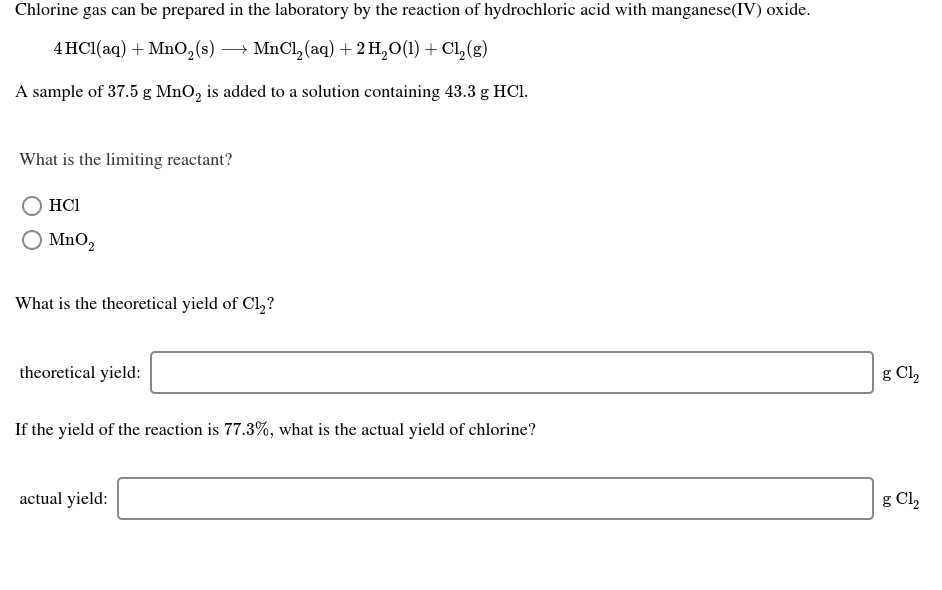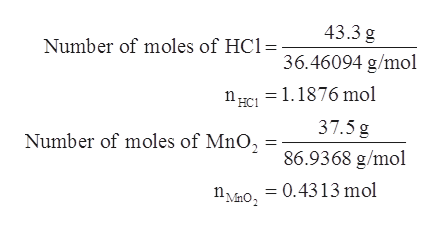# Chlorine gas can be prepared in the laboratory by the reaction of hydrochloric acid with manganese(IV) oxide.→ MnCl, (aq) + 2 H,0(1) + CL,(g)4 HC1(aq) + Mn0,(s)—A sample of 37.5 g MnO, is added to a solution containing 43.3 g HCI.What is the limiting reactant?HCIO MnO,What is the theoretical yield of Cl,?theoretical yield:g Cl,If the yield of the reaction is 77.3%, what is the actual yield of chlorine?actual yield:g Cl,

Question
4 views

helphelp_outlineImage TranscriptioncloseChlorine gas can be prepared in the laboratory by the reaction of hydrochloric acid with manganese(IV) oxide. → MnCl, (aq) + 2 H,0(1) + CL,(g) 4 HC1(aq) + Mn0,(s) — A sample of 37.5 g MnO, is added to a solution containing 43.3 g HCI. What is the limiting reactant? HCI O MnO, What is the theoretical yield of Cl,? theoretical yield: g Cl, If the yield of the reaction is 77.3%, what is the actual yield of chlorine? actual yield: g Cl, fullscreen
check_circle

Step 1

Given information:

Mass of MnO2 = 37.5 g

Mass of HCl = 43.3 g

Step 2

The chemical equation for the liberation of chlorine gas is represented as follows:

Step 3

First number of moles of HCl and MnO2 a...help_outlineImage Transcriptionclose43.3 g Number of moles of HC1= 36.46094 g/mol nci = 1.1876 mol 37.5 g Number of moles of MnO, 86.9368 g/mol nMmo, = 0.4313 mol fullscreen

### Want to see the full answer?

See Solution

#### Want to see this answer and more?

Solutions are written by subject experts who are available 24/7. Questions are typically answered within 1 hour.*

See Solution
*Response times may vary by subject and question.
Tagged in

### General Chemistry